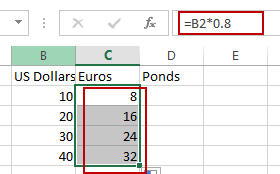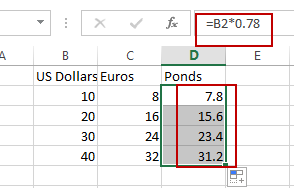# How to Convert Currency (USD,Euros,Punds) in Excel

This post will guide you how to convert from a given currency to other currencies in Excel. How do I convert US Dollars to the Euros with a formula in Excel.

## Convert US Dollars to Euros

If you want to convert currency from US Dollars to Euros in Excel, you need to know the latest exchange rate between USD and Euros. And Assuming that he current exchange rate is: 1 US Dollar =0.8 Euros. So we can use the following formula to convert currency from US Dollar to Euros.

=B2 * 0.8

Type this formula into blank cell and press Enter key to convert it. And then drag the AutoFill Handle over other cells to apply this formula.## Convert US Dollars to Pounds

If you need to convert from a given US dollars to pounds currency, you can use the following formula:

Assuming that the current exchange rate is: 1 US dollar=0.78.

=B2*0.78

Type this formula into blank cell and press Enter key to convert it. And then drag the AutoFill Handle over other cells to apply this formula.